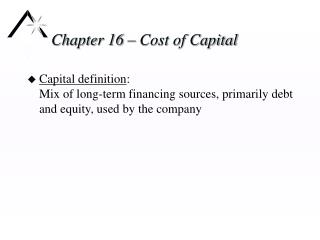# Chapter 16 – Cost of Capital - PowerPoint PPT PresentationDownload PresentationChapter 16 – Cost of Capital

Chapter 16 – Cost of Capital
Download Presentation## Chapter 16 – Cost of Capital

- - - - - - - - - - - - - - - - - - - - - - - - - - - E N D - - - - - - - - - - - - - - - - - - - - - - - - - - -
##### Presentation Transcript

1. Chapter 16 – Cost of Capital • Capital definition:Mix of long-term financing sources, primarily debt and equity, used by the company

2. Opportunity Cost Concept • Cost of capital is an opportunity cost • The opportunity cost is the return investors could have expected by investing somewhere else at equal risk

3. Weighted Average Concept • The cost of capital is an average of the opportunity costs of stockholders and creditors • The average is weighted by the proportion of funds provided by each source

4. Marginal Cost Concept • The marginal cost of capital is the rate of return that must be earned on new capital to satisfy investors • The marginal cost of capital is the weighted average cost of capital that must be earned on new investments • The marginal cost of capital is the change in the amount needed to satisfy investors, divided by the amount of new capital raised

5. Cost of Debt • Cost of debt is the interest rate the company would be required to pay on new debt, adjusted upward for flotation costs • Yield to maturity on bonds frequently measures the marginal cost of debt • To sell new bonds, you must pay at least the yield to maturity for new bonds • To justify reinvesting existing funds, your internal investments must be better than the return earned by buying back your existing debt in the marketplace

6. After-tax Cost of Debt • Payment of interest results in a tax savings • After-tax cost of debt:Kd = before-tax cost of debt X (1 – Tax rate)

7. Cost of Preferred Stock • Most preferred stock is perpetual; it has no maturity date • Cost of preferred stock:Kp = Dividend per share  Market price per share • The cost of new preferred stock requires the use of a net price, the amount that the company will net after paying flotation costs

8. Cost of Common Stock • No direct way to observe the return required by common stockholders; must estimate • Use • Dividend growth model • Earnings yield • CAPM

9. Dividend Growth Model • Ke = (D1/P) + gWhereD1 = Expected dividend at the end of the next year P = Current price of the stock g = Anticipated growth rate of dividends • Applies when dividend growth is stable

10. Earnings Yield Model • Ke = Earnings per share  Price • Sometimes used, but • Ignores growth • Not based on cash flow

11. Mean-variance CAPM • Ke = Rf + s,m[E(Rm) - Rf] Where Rf = Risk-free rate s,m = Beta of the company’s stock with regard to the market portfolio E(Rm) = Expected return on the market portfolio • Widely used • E(Rm) is still widely debated • Can use similar company betas for a stock that is not publicly traded

12. Cost of Existing Equity • Also called the cost of retained earnings • Some authors argue that the cost of retained earnings is Ke(1 – stockholders’ tax rate on dividends) • Much stock is held by pension funds and charitable endowments that do not pay taxes on dividends

13. Cost of new Equity • No dividend growth anticipated:Kne = Ke/(1 – f)where f is flotation cost as a percent of market price • With dividend growth anticipated:Kne = D1/[P/(1 – f)] + g

14. Weight for Weighted Average Cost • Market value weights are recommended • Target capital structure is typically used if the the company is moving toward that target

15. Additional Issues • Deferred taxes: typically not considered a source of funds because deferred tax reconciles difference between accounting records and cash flows • Accounts payable and accrued expenses: typically not considered a source of funds because they reconcile the difference between accounting and cash flow • Short-term debt is included if it is used as permanent capital

16. Additional Issues • Leases: often used as a direct substitute for debt, and therefore the implied amount and cost of debt is included in the WACC calculation (covered in Ch. 21) • Convertibles: treat as a straight bond and an option • Depreciation-generated funds: It makes sense to talk about internally generated funds, which have the cost of existing capital, but not to look separately at depreciation

17. Risk Differences and WACC • The WACC calculations typically assume that projects for a company are all of similar risk • When risks are different, they often vary by division • Companies often use division cost of capital, estimating the betas and cost of equity for each division if it were a stand-alone company • Large individual projects are sometimes evaluated as if they were funded as a stand-alone company

18. International Investments • Cost of capital should reflect the risk of the project • Cost of capital should be denominated in the same currency used to denominate cash flows for capital investment analysis • Equity residual method, discussed in Chapter 20, is sometimes used to evaluate international investments with multi-country financing.